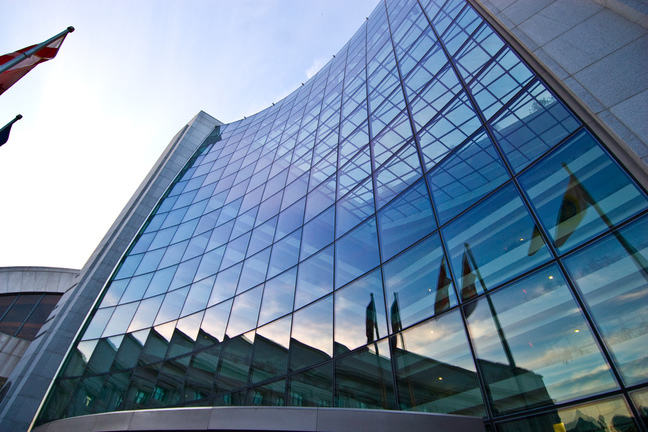## Want to keep learning?

This content is taken from the SOAS University of London's online course, Risk Management in the Global Economy. Join the course to learn more.
1.13

## SOAS University of LondonSecurities and Exchange Commission, Washington DC

# The return on bonds

There are two main measures of return on bonds: the current yield and the yield to maturity.

### Current Yield

The current yield, also known as interest yield or flat yield, is computed as the annual coupon payment divided by the market price of the bond.

For example, if a bond has a face value of $100, an annual coupon of 4% (that is,$4 is paid each year) and the bond is traded at a price of $90, its current yield is: $\frac {\4}{\90} = 0.0444 = 4.44\%$ From this can you see the relation between changes in the market price of bonds and bond yields? For example, what happens to the current yield on this bond if the market price of the bond falls? ### Yield to Maturity The previous step showed how the market price of a bond is related to the discounted future cash flows associated with the bond (the coupons and the repayment of principal on maturity): we discount the future cash flows to the present, and sum the discounted values. From that relation we can identify another measure of return on bonds, the yield to maturity. The yield to maturity, also known as redemption yield, is the rate of return y for which the current price of the bond, P, is equal to the present discounted value of the future cash flows, C(1), C(2),…, C(T): $P = \frac {C(1)}{(1+y)} + \frac {C(2)}{(1+y)^2} + ... + \frac {C(T)}{(1+y)^T}$ where C(1) is the cash flow in period 1, C(2) is the cash flow in period 2, and C(T) is the cash flow at maturity. For instance, if a bond pays an annual coupon payment of$20, a principal of $1,000 in two years, and is currently traded at the price$980, its yield to maturity is obtained by solving the following equation for $$y$$:

$\980 = \frac {\20}{(1+y)} + \frac {\20 + \1,000}{(1+y)^2}$

The solution of the above equation is $$y$$ = 0.0305. The yield to maturity of the bond is therefore 3.05%. Notice how the yield to maturity (3.05%) is higher than the coupon interest rate on the bond (2%). This is because at a market price of $980 you would pay less to buy the bond (which gives you the right to receive the coupon payments, and the face value at maturity) than the face value of the bond. We can ask the same question as before: what does this tell us about the relation between the market price of bonds and bond yields? For example, suppose you wanted a yield to maturity higher than 3.05%. Would you be prepared to pay more than$980 for this bond, or less than \$980?